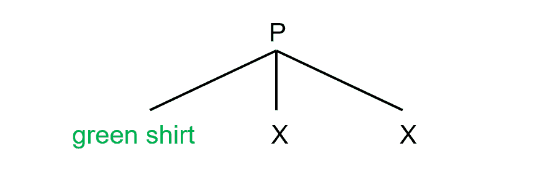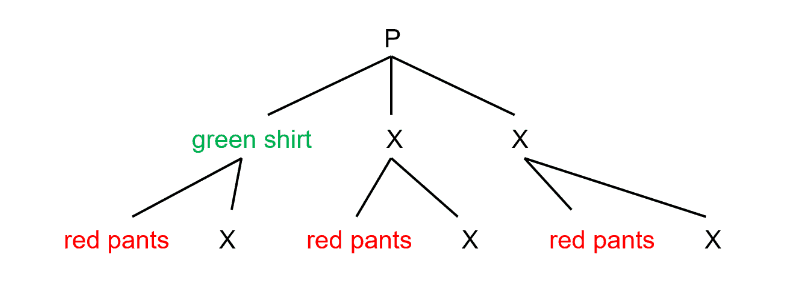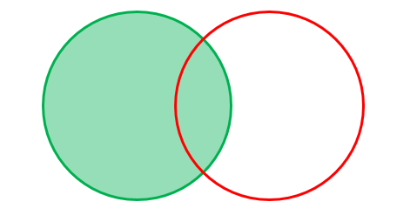# Deciphering the Probability of Multiple Events

by Sophia Stone

When solving probability problems, the how’s and why’s are often just as important as the calculations themselves. Half the battle is decoding the question stem and recognizing the type of probability being tested. Fortunately, there are some patterns inherent to the language of probability that can help you recognize the type of probability being tested right away so you know exactly what formula to use.

## If you see AND, then you should think MULTIPLICATION.

Example: What is the probability that I wore a green shirt and red pants?

Despite the fact that the wearer might not have the best fashion sense, let’s say that the wearer has three different shirts, so the  probability of randomly selecting a green shirt is ⅓. The probability of selecting red pants we will assume is entirely independent of the shirt chosen – wearing a green shirt makes the wearer no more or less likely to wear red pants. Let’s say the wearer only has two pairs of pants, so the probability of randomly selecting red pants is ½. The probability of two independent events occurring simultaneously is the product of their probabilities. Let’s prove this by drawing a tree diagram. There are three different shirt options:For every shirt option, the wearer has two pairs of pants to choose from:Therefore, there are six possible shirt-pant combinations, and only one of them is the one we are interested in. The probability of selecting a green shirt (⅓) and red pants (½) is the product of their probabilities: 1/3 × 1/2 = 1/6.

## If you see OR, then you should think ADDITION.

Example: What is the probability that I wore a green shirt or red pants?

Well, what exactly is meant by this? Does this mean situations where the wearer wears at least a green shirt or red pants, like so:Or does this mean situations where the wearer wears either a green shirt or red pants, but not both, like so:Well, it entirely depends on the language of the question stem! The word “or” by itself means “one or the other or both.” The question has to specify “but not both” if that’s what they want. We can clearly see that there is a 4/6 or 2/3 probability of wearing at least a green shirt or red pants. How should we prove this mathematically?

Well, let’s take all the green shirt combinations:Then let’s add any red pants combinations:Do you see a problem here? We’ve double-counted the green shirt-red pants combination! For this reason, when we find the probability of at least one or another event occurring, we must add the probability of each event together and then subtract the probability of them occurring simultaneously – because otherwise we’ll be counting that twice!

### P (at least A or B) = P(A) + P(B) – P(A and B)

Remember that the probability of both occurring simultaneously is found by multiplying the two probabilities together. For this example, we would get:Another way to look at this is to use a Venn diagram. Let’s say the green circle represents all possibilities that include a green shirt, and the red circle represents all possibilities that include red pants. The overlapping region represents all possibilities that include both a green shirt and red pants.Like before, first we’ll consider all the green shirt combinations. Notice how this counts combinations with both a green shirt and red pants:Now let’s consider all the red shirt combinations. Uh-oh, we’ve counted combinations with both a green shirt and red pants again!To account for this, when solving for the probability of at least one event or another event, we must add the probability of each event occurring, and then subtract the probability of both occurring simultaneously – because we’ve counted that overlapping region of the Venn diagram twice!

Now let’s return to a scenario where you are asked for the probability of wearing either a red shirt or green pants (but not both). This is where critical thinking comes in. Well, we can start by calculating the probability of wearing at least a green shirt or red pants, as we just did, which is 2/3. Then we need to subtract the probability of both occurring simultaneously again (1/3 × 1/2 = 1/6):You are less likely to see the last example of a probability problem compared to more classic examples of probabilities problems on the PCAT. However, the key takeaways from this example are that it is critical to stay alert, and incorporating critical reasoning strategies is often just as important as recalling the right formula on Test Day. Sometimes all you will need is the right equation to solve a probability question. Other times, it’s smarter to jot down or sketch out all possible scenarios and count it out. But ultimately, the more you practice and expose yourself to all sorts of probability problems, the less likely you are to be surprised by anything you see on Test Day!

Good luck!

#### Submit a Comment

This site uses Akismet to reduce spam. Learn how your comment data is processed.